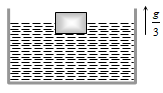NEET Physics Mechanical Properties of Fluids Questions Solved

A cubical block is floating in a liquid with half of its volume immersed in the liquid. When the whole system accelerates upwards with acceleration of g/3, the fraction of volume immersed in the liquid will be(a) $\frac{1}{2}$                                  (b) $\frac{3}{8}$

(c) $\frac{2}{3}$                                  (d) $\frac{3}{4}$

Explanation is a part of a Paid Course. To view Explanation Please buy the course.

Difficulty Level: